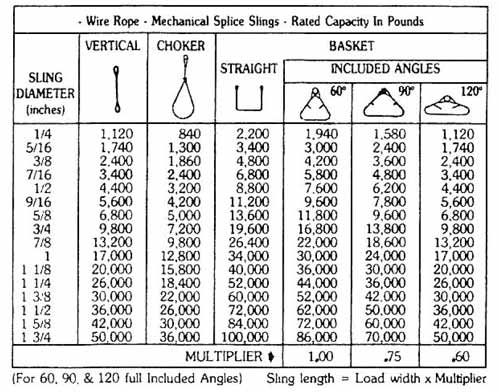Mike ParnellOct 29, 2010 9:52:00 AM2 min read

# Calculating Sling Legs

Calculating Sling Legs

When our instructors conduct rigging courses, they teach the participants a quick method to calculate the minimum size of length of slings for a given load using a formula which has been included at the bottom of our rated capacity card.

Problem:  We need to install a set of slings between the crane hook and a spreader bar, which will create a 90 degree full included angle or less.

Question #1:  What size of wire rope slings do we need?

Facts:  Spreader bar length is 8’.  The weight of the load, bar and rigging gear will be 12,000 lbs.

Solution #1:  Since we want to rig the load at a 90 degree angle or less, we can look in the 90 degree column on the rated capacity card and find what size of wire rope meets or exceeds 12,000 lbs. We find that the sling legs will need to be made of at least ¾” wire rope.

Question #2:  What is the minimum length for these slings?

Facts:  A quick calculating formula has been included at the bottom of the rated capacity card.

SLING LENGTH is the minimum length of sling needed from the crane hook to the sling’s connection or contact point on the load.

MULTIPLIER is the number located at the bottom of each degree angle column, and is used in the formula according to which angle (60, 90, 120) the slings form when installed.

Sling Length = Load Width x Multiplier

Solution #2:  Since the pad eyes are 8’ apart, let 8 represent LOAD WIDTH and the MULTIPLIER is .75, as taken from the bottom of the 90 degree column.

SLING LENGTH = 8’ x .75

SLING LENGTH = 6’

Now a chance to test the reader’s skills.

What size and length of slings would you need between the hook and a 20’ spreader bar?  (Total weight of load and rigging = 25,000 lbs)

Angle                  Size                           Length

60                      ______         x         ______

90                      ______         x         ______

120                    ______         x         ______

(Solution is below, so don't scroll too far unless you want to see the answers).

Solution:

Angle            Size            Length

60                1”                x  20’

90                1 1/8”          x  15’

120              1 ¼”            x  12’

Happy Rigging, Mike Parnell.  Click here to order the Journeyman Rigger's Reference Card, which is utilized in this workshop.

This is an article from The Professional Rigger newsletter, 1988.